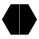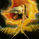# Fractal Support Resistance (Fixed Volume) 2

צפיות ‎20280‎
20280
Fractal Support Resistance (Fixed Volume ) 2

http://synapticex.com
```//@version=2
//synapticex.com
study("Fractal Support Resistance", shorttitle="FSR", overlay=true)
tf = input(title="Resolution", type=resolution, defval = "current")
vamp = input(title="VolumeMA", type=integer, defval=6)
vam = sma(volume, vamp)

up = high>high and high>high and high<high and high<high and volume>vam
down = low<low and low<low and low>low and low>low and volume>vam
fractalup =  up ? high : fractalup
fractaldown = down ? low : fractaldown

fuptf = security(tickerid,tf == "current" ? period : tf, fractalup)
fdowntf = security(tickerid,tf == "current" ? period : tf, fractaldown)

plot(fuptf, "FractalUp", color=lime, linewidth=1, style=cross, transp=0, offset =-3, join=false)
plot(fdowntf, "FractalDown", color=red, linewidth=1, style=cross, transp=0, offset=-3, join=false)

```

## תגובותTonyCampbell
lol absolutely. If you find an error let me know. Thanks.
השבsynapticEx
OK......great, thanks. I googled this S/R for background info....articles etc, but didn't find much......do you know of anything?
השבsynapticEx
Does it seem to work best for a particular TF.....intraday or swing trading over multi days or weeks? A great addition, thanks again !
השב